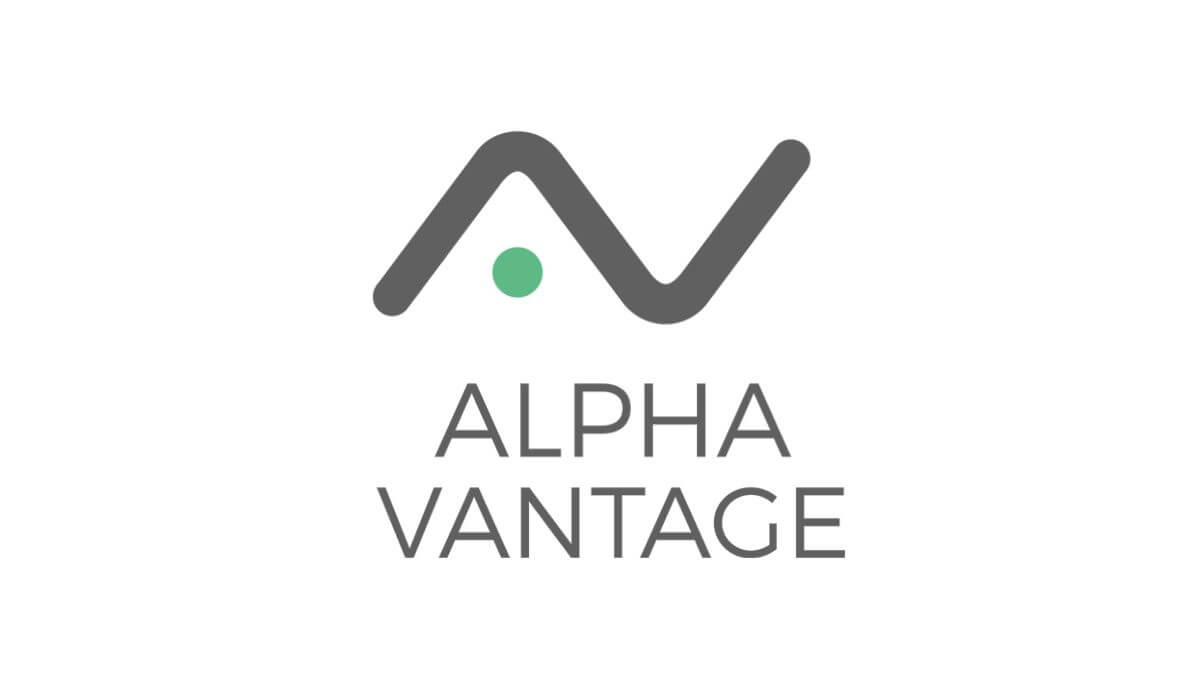# Alpha Vantage 获取实时美股及数字货币数据

Alpha Vantage 是一个能够让你通过 Json 和 Pandas DataFrame 格式获取免费实时金融数据的API。

https://www.alphavantage.co/support/#api-key

## 1.准备

(可选1) 如果你用Python的目的是数据分析，可以直接安装Anaconda：Python数据分析与挖掘好帮手—Anaconda，它内置了Python和pip.

(可选2) 此外，推荐大家用VSCode编辑器来编写小型Python项目：Python 编程的最好搭档—VSCode 详细指南

Windows环境下打开Cmd(开始—运行—CMD)，苹果系统环境下请打开Terminal(command+空格输入Terminal)，输入命令安装依赖：

```pip install alpha_vantage
```

## 2.基本使用

```from alpha_vantage.timeseries import TimeSeries
ts = TimeSeries(key='你的API Key')
print(data)```

```from alpha_vantage.timeseries import TimeSeries
ts = TimeSeries(key='你的API Key', output_format='pandas', indexing_type='date')
print(data)```

```from alpha_vantage.timeseries import TimeSeries
ts = TimeSeries(key='你的API Key', output_format='pandas', indexing_type='date')
data, meta_data = ts.get_intraday('OXY', interval='1min', outputsize='full')
print(data)```

## 3.高级功能

```import asyncio
from alpha_vantage.async_support.timeseries import TimeSeries

symbols = ['AAPL', 'GOOG', 'TSLA', 'MSFT']

async def get_data(symbol):
ts = TimeSeries(key='YOUR_KEY_HERE')
data, _ = await ts.get_quote_endpoint(symbol)
await ts.close()
return data

loop = asyncio.get_event_loop()
tasks = [get_data(symbol) for symbol in symbols]
results = loop.run_until_complete(group1)
loop.close()
print(results)```

​Python实用宝典 ( pythondict.com )